# JP3675014B2 - Inverter control device - Google Patents

## Info

Publication number
JP3675014B2
JP3675014B2 JP01428796A JP1428796A JP3675014B2 JP 3675014 B2 JP3675014 B2 JP 3675014B2 JP 01428796 A JP01428796 A JP 01428796A JP 1428796 A JP1428796 A JP 1428796A JP 3675014 B2 JP3675014 B2 JP 3675014B2
Authority
JP
Japan
Prior art keywords
torque
means
magnetic flux
primary magnetic
primary
Prior art date
Legal status (The legal status is an assumption and is not a legal conclusion. Google has not performed a legal analysis and makes no representation as to the accuracy of the status listed.)
Expired - Fee Related
Application number
JP01428796A
Other languages
Japanese (ja)
Other versions
JPH0956195A (en
Inventor

Original Assignee

Priority date (The priority date is an assumption and is not a legal conclusion. Google has not performed a legal analysis and makes no representation as to the accuracy of the date listed.)
Filing date
Publication date
Priority to JP14222495 priority Critical
Priority to JP7-142224 priority
Application filed by 株式会社デンソー filed Critical 株式会社デンソー
Priority to JP01428796A priority patent/JP3675014B2/en
Publication of JPH0956195A publication Critical patent/JPH0956195A/en
Application granted granted Critical
Publication of JP3675014B2 publication Critical patent/JP3675014B2/en
Anticipated expiration legal-status Critical
Application status is Expired - Fee Related legal-status Critical

## Images

•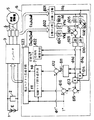•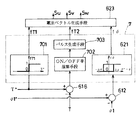•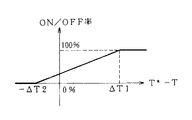••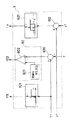•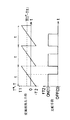•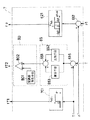••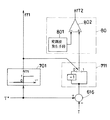•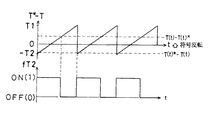•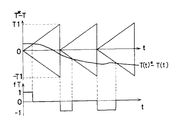••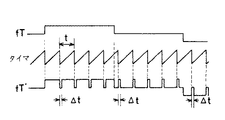•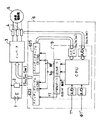•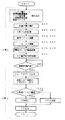•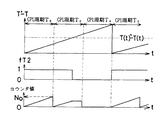•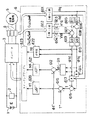•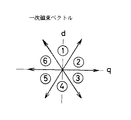•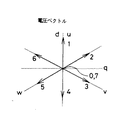## Classifications

• HELECTRICITY
• H02GENERATION; CONVERSION OR DISTRIBUTION OF ELECTRIC POWER
• H02PCONTROL OR REGULATION OF ELECTRIC MOTORS, ELECTRIC GENERATORS OR DYNAMO-ELECTRIC CONVERTERS; CONTROLLING TRANSFORMERS, REACTORS OR CHOKE COILS
• H02P21/00Arrangements or methods for the control of electric machines by vector control, e.g. by control of field orientation
• H02P21/06Rotor flux based control involving the use of rotor position or rotor speed sensors
• H02P21/08Indirect field-oriented control; Rotor flux feed-forward control
• H02P21/09Field phase angle calculation based on rotor voltage equation by adding slip frequency and speed proportional frequency
• HELECTRICITY
• H02GENERATION; CONVERSION OR DISTRIBUTION OF ELECTRIC POWER
• H02PCONTROL OR REGULATION OF ELECTRIC MOTORS, ELECTRIC GENERATORS OR DYNAMO-ELECTRIC CONVERTERS; CONTROLLING TRANSFORMERS, REACTORS OR CHOKE COILS
• H02P21/00Arrangements or methods for the control of electric machines by vector control, e.g. by control of field orientation
• H02P21/22Current control, e.g. using a current control loop

## Description


BACKGROUND OF THE INVENTION
The present invention relates to a method for controlling torque vector of an induction motor by controlling a voltage vector of a PWM inverter, and relates to an inverter control device capable of operating with low frequency magnetic noise by operating an inverter switching frequency in a desired band. In addition, the present invention relates to an inverter control device with good torque response.

[Prior art]
Conventionally, as a method for controlling torque of an induction motor at high speed, “New High Speed Torque Control Method for Induction Motor Based on Instantaneous Slip Frequency Control” on Vol.
FIG. 17 shows a block diagram of the torque control method described in the paper. In this block diagram, 1 is a battery, 2 is a voltage detector of the battery 1, and 3 is a PWM inverter, which is composed of six arms each composed of a switching element such as a transistor and a diode connected in reverse parallel. It can be expressed as a single switch.
4 is a current detector, 5 is a three-phase induction motor, and 6 is an inverter control circuit.

In the inverter control circuit 6, 601 is a block for converting the primary current vectors of the three-phase currents iu, iv, iw detected by the current detector 4 into biaxial components i1d, i1q of d, q, and 602 is an inverter control circuit 3. A block for calculating a unit vector of the primary terminal voltage from the switching states (Su, Sv, Sw) of the inside, and 603 and 604 multiply the unit vector by the battery voltage detected by the voltage detector 2, and each axis of the primary terminal voltage vector This is a block for calculating the components V1d and V1q.
Reference numerals 605 and 606 denote blocks for calculating a voltage value by multiplying the biaxial components i1d and i1q of the primary current vector of the three-phase current by the primary winding resistance value R1 of the induction motor 5, and reference numerals 607 and 608 denote primary values obtained by the blocks 603 and 604, respectively. Blocks 609 and 610 subtract the voltage values of blocks 605 and 606 from the axis components V1d and V1q of the terminal voltage. 609 and 610 integrate the voltage values of blocks 607 and 608, thereby calculating the axis components φ1d and φ1q of the primary magnetic flux vector. Is a block for calculating.

Reference numeral 611 denotes a block for calculating the primary magnetic flux vector length φ1 from the respective axial components φ1d and φ1q of the primary magnetic flux vector, and reference numeral 612 denotes a primary magnetic flux deviation Δφ by subtracting the primary magnetic flux vector length φ1 from the primary magnetic flux command value φ1 *. The obtained blocks 613 and 614 calculate the product of the biaxial components i1d and i1q of the primary current vector from the block 601 and the axial components φ1d and φ1q of the primary magnetic flux vector from the blocks 609 and 610 to calculate the respective axial components of the instantaneous torque T. 615 is a block for obtaining the instantaneous torque T from each axis component obtained by the blocks 613 and 614, and 616 is a block for obtaining the torque deviation ΔT by subtracting the instantaneous torque T from the torque command value T *.

Reference numeral 620 denotes a three-value hysteresis comparator, which has a predetermined torque deviation ΔT between the instantaneous torque T calculated as the vector product of the primary magnetic flux vector and the motor current and the torque command value T * according to the torque deviation ΔT obtained in block 616. A torque flag fT indicating a current torque holding command is generated when the torque is within the range, and an increase / decrease command is generated when exceeding the predetermined range.
Reference numeral 621 denotes a binary hysteresis comparator. When the length φ1 of the primary magnetic flux vector calculated in block 611 is larger than the primary magnetic flux command value φ1 * in accordance with the primary magnetic flux deviation Δφ obtained in block 612, the primary magnetic flux is indicated. The primary magnetic flux flag fφ representing the primary magnetic flux increase command is generated when the command is small.
622 is a vector phase determination block, and the phase angle θ of the primary magnetic flux vector is 30 °, 90 °, 150 °, 210 °, 270 °, 330 ° in the clockwise direction with respect to the d-axis as shown in FIG. It is determined which of the six areas {circle around (1)} to {circle around (6)} divided every 60 °, and a phase flag fθ corresponding to the belonging area is generated.

Reference numeral 623 denotes a switching table constituted by a ROM, which stores a voltage vector with optimized torque response. Here, eight patterns shown in Table 1 are stored. When a torque flag fT, a primary magnetic flux flag fφ, and a phase flag fθ are given, the PWM inverter 3 corresponding to an optimum voltage vector corresponding to these flags. The switching pattern is output.
Each numerical value in Table 1 indicates the direction of each voltage vector indicated by the numerical value in FIG.

[Table 1]

With the above configuration, the inverter control circuit 6 calculates the primary magnetic flux vector using the voltage vector output from the switching table 623, the battery voltage, the motor current, and the motor primary winding resistance value, and changes the switching pattern accordingly. Determine and output to the PWM inverter 3.
On the other hand, in Japanese Utility Model Publication No. 6-26079, the hysteresis value of the hysteresis comparator that generates the advance and stop of the phase of the primary magnetic flux vector so that the torque follows the given torque command value within a predetermined error. When a low torque command value is given, means for converting the torque command value into an on / off pulse train having an amplitude equal to the hysteresis value is provided in the above configuration to reduce the start-up of the motor and the impact of the shift.

[Problems to be solved by the invention]
As described above, the above-described conventional technique is a method of reading a voltage vector stored in advance in the ROM according to the control flag (torque flag fT, primary magnetic flux flag fφ, phase flag fθ) every moment. Since this time interval appears as a result of calculation in each block, the time interval until the voltage vector transitions to the next state becomes indefinite, and the switching frequency of the PWM inverter cannot be set to a predetermined value.
For this reason, for example, when the switching frequency of the PWM inverter is lower than a predetermined value, the frequency component of the audible region included in the primary winding current of the motor increases, so that annoying magnetic noise from the motor is generated. Problems such as increased torque fluctuation due to large distortion of the current flowing through the motor, and the inverter's overcurrent protection function being activated due to unexpectedly large current flowing through the motor, which prevents smooth operation. There is.
Further, due to the influence of the counter electromotive force generated inside the motor, the average value of the instantaneous torque with respect to the torque command value decreases as the rotational speed increases, and thus there is a problem that the output torque decreases as the rotational speed increases.
Further, in the above publication, since the pulse width modulation operation is only performed when the torque command value is lower than the hysteresis value of the hysteresis comparator, these problems cannot be solved.

An object of the present invention is to provide an inverter control device that is small in size and has little magnetic noise and torque fluctuation by setting the switching frequency of an inverter to a desired value with a simple configuration.

[Means for Solving the Problems]
The present inventionIn claim 1 ofWhen the primary terminal voltage applied to the multiphase induction motor and the primary current flowing through the multiphase induction motor are detected by the voltage detection means and the current detection means, respectively,,The primary magnetic flux vector and the instantaneous torque value are respectively calculated by the magnetic flux vector calculating means and the torque calculating means, and the voltage vector based on the deviation between these calculated values and the primary magnetic flux command value and the torque command value and the phase angle of the primary magnetic flux vector. Is generated by the voltage vector generating means.
Since the voltage vector generating means for generating the voltage vector includes voltage vector control means for controlling the transition of the voltage vector, the voltage vector transition can be changed to a desired period regardless of the calculation period of the primary magnetic flux vector and the instantaneous torque value. Can be.
Therefore, by limiting the switching speed in the inverter and controlling the upper limit of the switching frequency, the inverter cooling can be suppressed to reduce the size of the inverter cooling device, or the voltage vector can be forced at fixed time intervals. By changing to a different voltage vector, the switching frequency can be increased, and by limiting the lower limit of the switching frequency, it is possible to prevent the occurrence of magnetic noise and torque fluctuation of the motor.
Specifically, since the voltage vector control means has a limiting means for limiting the change of the voltage vector for a predetermined period, the switching speed in the inverter is limited to set the upper limit of the switching frequency, thereby suppressing the heat generation of the inverter. Thus, the inverter cooling device can be downsized.
In addition, since the voltage vector control means has an operation means for operating the generated voltage vector to a different voltage vector at an arbitrary time interval, the voltage vector is forcibly changed to a different voltage vector at a fixed time interval. As a result, the switching frequency can be increased. Thereby, by lowering the switching frequency and limiting the lower limit of the switching frequency, it is possible to set the desired switching frequency and prevent the occurrence of magnetic noise and torque fluctuation of the electric motor.

Claim 2The primary magnetic flux is calculated from the primary voltage applied to the multiphase induction motor and the primary current flowing through the multiphase induction motor.vectorAnd instantaneous torquevalueIs calculated. Primary magnetic flux command value and primary magnetic fluxvectorofPrimary magnetic fluxDeviation, torque command value and instantaneous torquevalueControl flag from deviation fromDecisionThen, a voltage vector to be output to the inverter is generated based on the control flag and the phase angle of the primary magnetic flux vector.
A control flag group generating means for generating a pulse generating means for generating a pulse for repeatedly turning on and off the control flag while a predetermined time elapses;HaveBy a given timeButVoltage vector every timeButBecause it is changed, the primary magnetic flux vector and instantaneous torquevalueRegardless of the calculation cycle, the transition of the voltage vector can be set to a desired cycle. That is, the upper limit of the switching frequency in the inverter, depending on the pulse periodandThe lower limit is limitedTheThatSwitchingBy limiting the upper frequency limit,It is possible to reduce the size of the inverter cooling device by suppressing the heat generation of the inverter.The AlsoBy limiting the lower limit of the switching frequency, it is possible to prevent the occurrence of magnetic noise and torque fluctuation of the motor.

Claim 3Let's set the control flag every predetermined time,By providing changing means for changing to a different value for a time shorter than the predetermined time, the voltage vector isButBecause it is changed, the primary magnetic flux vector and instantaneous torquevalueRegardless of the calculation cycle, the transition of the voltage vector can be set to a desired cycle. In other words, the lower limit of the switching frequency in the inverter is limited, preventing the occurrence of magnetic noise and torque fluctuations in the motor.To doit can.

Claim 4Then, by applying the zero voltage vector to the voltage vector operated by the pulse generating means or changing means, the rapid change of the primary current is suppressed.To doCan prevent motor torque fluctuationsTo doit can.

Claim 5Then, the modulated wave generating means generates a modulated wave that repeatedly increases and decreases alternately with a predetermined amplitude, and the pulse width modulating means generates a modulated wave based on the modulated wave.Primary flux deviation or torquePerform pulse width modulation of the deviation. When a small torque command value is given at the time of starting or operating the motor, the average voltage vector is generated as a small value compared to the case where the conventional control is performed, so that the shock at the time of starting the motor is reduced.Benefits.

Claim 6Then, when performing pulse width modulation,Primary flux deviation or torqueWhen the compensation signal with proportional or proportional integral amplification of the deviation is subjected to pulse width modulation based on this modulation wave,torqueControl with less command value errorTheRealizationTo doit can.
Claim 7Then, the limiter means to cross the modulated wave, Primary magnetic flux deviation, torqueDeviation ortheseWhen pulse width modulation is performed with the compensation signal size limited, the voltage is changed to a different voltage vector within the period of the modulated wave.InControls the lower limit of switching frequencyCan beThe

Claim 8Then, based on the operating quadrant of the multiphase induction motor by the sign operating meansPrimary flux deviation or torqueDeviation orthesePulse width modulation is performed by operating one of the codes of the compensation signal or the modulated waveBy4 quadrant operation of the motorPossibleThe

In claim 9Detects the time when the pulse generating means or changing means operates the control flag, and based on this time, the primary voltage is detected.ofCorrecting the calculated value to the actual valueVoltageCorrection means are provided. This manipulated the voltage vector to a different valueIf the motorEven if an error occurs between the actual value of the torque or primary magnetic flux vector and the calculated value, a voltage vector that takes into account the error is output.LetCan.

DETAILED DESCRIPTION OF THE INVENTION
Next, the present invention will be described based on embodiments shown in the drawings.
FIG. 1 shows an inverter control apparatus according to a first embodiment of the present invention. In the figure, what is denoted by the same reference numerals as those of the prior art in FIG. The voltage vector generation means 623 is equivalent to the switching table 623 in the prior art.
In the present invention, the voltage vector control means 7 is provided in the corresponding part of the ternary hysteresis comparator 620 and the binary hysteresis comparator 621 in the prior art.

In the prior art of FIG. 17, the torque flag fT that is the output of the ternary hysteresis comparator 620 is a control flag that has one of three states of torque increase / decrease and current status as is well known. Therefore, in the first embodiment, the torque flag fT is used as a control flag for instructing whether to increase the torque in the forward or reverse direction of the induction motor 5, and the torque increase. -It is expressed as two torque flags including a second torque flag fT2 as a control flag for instructing to maintain the current state.
Table 2 shows the correspondence with the torque flag fT in the conventional control.

[Table 2]

As shown in FIG. 2, the control flag generation means (voltage vector control means) 7 according to the first embodiment determines the polarity of the torque command value and outputs the first torque flag fT1 to the voltage vector generation means 623. The polarity discriminating means 701, the ON / OFF rate calculating means 702 for calculating the ON / OFF rate from the torque deviation, the ON / OFF pulse is generated according to the ON / OFF rate, and the voltage vector generating means 623 receives the second torque flag fT2. And a binary hysteresis comparator 621 are provided.

FIG. 3 shows the operation of the ON / OFF rate calculating means 702 in the first embodiment.
The first torque flag fT1 is generated by the polarity discriminating means 701, and when the torque command value is positive (the induction motor is operated by normal rotation / power running or reverse rotation / regeneration), the normal rotation direction (three-value hysteresis in the prior art) The output of the comparator 620 is given to the voltage vector generation means 623 as a command fT1 = 1 for increasing the torque in the direction corresponding to “1”. On the other hand, the second torque flag fT2 is generated by the ON / OFF rate calculating means 702, and as shown in FIG. 3, 0% is output when the torque deviation is equal to or less than a threshold value Δ−T2, and when it is equal to or greater than ΔT1. Outputs 100%, and in the middle, outputs a value between 0 and 100%, and outputs the calculated ON / OFF rate to the pulse generation means. In FIG. 3, the ON / OFF rate changes linearly with respect to the torque deviation, but is not limited to a straight line and may be a curved line.

The pulse generation means 703 has a predetermined time t (seconds).
ON time (seconds) = t (seconds) × ON / OFF rate (%) / 100 (%)
OFF time (seconds) = t (seconds) × (100−ON / OFF rate (%) / 100 (%)
A pulse that repeats on / off at a rate of is generated. The generated pulse is given to the voltage vector generating means 623 as the second torque flag fT2.
In the above equation, ON corresponds to the second torque flag fT2 corresponding to 1 in Table 2, and OFF corresponds to the second torque flag fT2 corresponding to 0.

With the above configuration, in the first embodiment, unless the ON / OFF rate is 0% or 100%, the second torque flag fT2 generated during the predetermined time t (seconds) is repeatedly turned on and off, Since the voltage vector is updated at least twice during t (seconds), the transition of the voltage vector can be set to a desired period regardless of the calculation period of the primary magnetic flux vector and the instantaneous torque. Accordingly, by limiting the upper limit of the switch frequency in the PWM inverter 3, it is possible to reduce the heat generation of the PWM inverter 3 and to reduce the size of the inverter cooling device, or by limiting the lower limit of the switching frequency, the induction motor 5 The occurrence of magnetic noise and torque fluctuations can be prevented.

As can be seen from Table 2, when the second torque flag fT2 is OFF, the voltage vector generation means 623 selects a zero voltage vector that is a voltage vector in which all line voltages of the induction motor 5 are zero. As in the above embodiment, on-off control of the second torque flag fT2 at a ratio corresponding to the torque deviation allows the zero voltage vector to be used frequently when the torque deviation is small, and conversely when the torque deviation is large. The voltage vector that increases the torque can be used extensively. Therefore, when a small torque command value is given at the time of starting or operating the induction motor 5, the average voltage vector is generated as a smaller value than when the conventional control is performed. Can be small. In addition, by using the zero voltage vector, it is possible to suppress a sudden change in the primary current and a sudden torque change generated in the induction motor 5, thereby reducing the impact of the motor.

In the first embodiment, in the control flag group generation means (voltage vector control means) 7, an ON / OFF rate calculation means 702 and a pulse generation means 703 are provided in the subsequent stage of the torque deviation calculation means 616 and a binary hysteresis comparator 621 is provided. Although provided in the subsequent stage of the primary magnetic flux deviation calculating means 612, in the control flag group generating means 7, although not shown, the ON / OFF rate calculating means 702 and the pulse generating means 703 are provided in the subsequent stage of the primary magnetic flux deviation calculating means 612. Even if the ternary hysteresis comparator 620 is provided in the subsequent stage of the torque deviation calculation means 616, the voltage vector is updated at least twice during t (seconds), so regardless of the calculation cycle of the primary magnetic flux vector and the instantaneous torque value. The transition of the voltage vector can be set to a desired period. In this configuration, the polarity discriminating means 701 is not necessary.

In the first embodiment, although not shown in the control flag group generation means 7, the ON / OFF rate calculation means 702 and the pulse generation means 703 are replaced with the subsequent stage of the torque deviation calculation means 616 and the primary magnetic flux deviation calculation means 612. Even if each is provided in the subsequent stage, the same effect as in the above embodiment can be obtained.
In the first embodiment, although not shown in the control flag group generation unit 7, the upper / lower limit values of the primary magnetic flux deviation or torque deviation or the ON / OFF rate are set in the ON / OFF rate calculation unit 702. By providing the limiting means for limiting, the torque flag fT2 generated during the predetermined time t (seconds) is always turned on and off, so that the primary magnetic flux deviation or torque deviation or the ON / OFF ratio is any value. The voltage vector is updated at least twice during t (seconds), and the magnetic noise and torque fluctuation of the induction motor 5 can be further reduced.
In the first embodiment, although not shown in the control flag group generation means 7, even if the polarity determination means 701 is provided in the subsequent stage of the torque deviation calculation means 616, the same effect as in the above embodiment is obtained.

In the first embodiment, the control flag group generation unit 7 generates each of the voltage vector generation units (switching tables) 623 and 624 using the pulses generated by the pulse generation unit 703 as shown in FIG. The configuration in which the voltage vector is switched and the voltage vector is output to the PWM inverter 3 has the same effect as the above embodiment.
The on / off cycle t of the second torque flag fT2 may be arbitrarily set by changing the time width t.

FIG. 5 shows a second embodiment of the present invention.
In the second embodiment, instead of the ON / OFF rate calculating means 702 and the pulse generating means 703 in FIG. 2, the pulse width modulating means 80 for converting the second torque flag fT2 into on / off pulses at a rate corresponding to the torque deviation. Is provided. The pulse width modulation unit 80 includes a modulation wave generation unit 801 that generates a modulation wave, and a comparison unit 802 that compares the modulation wave and the torque deviation and outputs a binary value of ON or OFF.

FIG. 6 shows the operation of the second embodiment in detail.
As shown in the upper part of FIG. 6, the modulated wave generation unit 801 outputs a triangular wave having a predetermined period t, a minimum value of −ΔT2, and a maximum value of ΔT to the comparison unit 802, for example. As shown in the lower part of FIG.
If modulation wave size> torque deviation, fT2 = ON
If modulation wave size <torque deviation, fT2 = OFF
Is output. Since the modulated wave repeats increasing and decreasing at the period t, the comparing means 802 outputs a pulse that repeats on and off at the period t. The generated pulse is given to the voltage vector generating means 623 as the second torque flag rfT2. In the above, ON corresponds to the second torque flag fT2 corresponding to “1” in Table 2, and OFF corresponds to the second torque flag fT2 corresponding to “0” in Table 2.

With the above configuration, the second embodiment has the same effects as the first embodiment. That is, it is possible to reduce the size of the inverter cooling device, to prevent the induction motor 5 from generating magnetic noise and torque fluctuations, or to reduce the impact during starting and operation of the motor.

In the second embodiment, the period t of the modulated wave (triangular wave) of the modulated wave generating means 801 may be variable. In the second embodiment, in the control flag group generation means 7, the pulse width modulation means 80 is provided in the subsequent stage of the torque deviation calculation means 616, and the binary hysteresis comparator 621 is provided in the subsequent stage of the primary magnetic flux deviation calculation means 612. Although not shown in the control flag group generation means 7, the pulse width modulation means 80 is provided at the subsequent stage of the primary magnetic flux deviation calculation means 612, and the ternary hysteresis comparator 620 shown in FIG. Even if it is provided in the subsequent stage of 616, the same effect as in the above embodiment is obtained.
In the second embodiment, although not shown in the control flag group generation means 7, the pulse width modulation means 80 may be provided in the subsequent stage of the torque deviation calculation means 616 and the subsequent stage of the primary magnetic flux deviation calculation means 612. There are the same effects as in the embodiment.

FIG. 7 shows a third embodiment of the present invention.
In the third embodiment, in the control flag group generation means 7 in the second embodiment shown in FIG. 5, the torque deviation is proportionally integrated between the subsequent stage of the torque deviation calculation means 616 and the previous stage of the pulse width modulation means 80. A proportional integration means 85 for outputting a compensation signal is added. The proportional integration unit 85 includes a proportional unit 852 that amplifies the torque deviation, an integration unit 853 that integrates the torque deviation, and an addition unit 851 that adds the outputs and outputs a compensation signal.

In the configuration shown in FIG. 7, the ratio between the ON time and the OFF time of the second torque flag fT2 is determined based on the value of the compensation signal output from the proportional integration means 85. Increasing the value of the compensation signal increases the ON time, resulting in an increase in torque. Conversely, decreasing the value of the compensation signal decreases the ON time, resulting in a decrease in torque.

FIG. 8 shows the operation of the third embodiment in detail.
The upper part of the figure shows how the second torque flag fT2 is repeatedly turned on and off at a predetermined period t, and the lower part of the figure shows how the instantaneous torque changes due to the second torque flag fT2. FIG.

The integrating means 853 stores and outputs the sum of the errors between the torque command value and the instantaneous torque, so that the ratio between the ON time and the OFF time of the second torque flag fT2 so that the torque command value and the average value of the instantaneous torque coincide with each other. Are operated sequentially. That is, the integration is continued until the sum of the areas of the hatched portion A and the hatched portion B in FIG. 8 becomes equal. Therefore, in the steady state c where the sum of the areas becomes equal, a compensation signal as an integration result is output. However, the torque command value and the average value of the instantaneous torque match.

The proportional means 852 sequentially operates the ratio between the ON time and the OFF time of the second torque flag fT2 so that the torque command value T * and the instantaneous torque T quickly coincide with each other. For example, in FIG. 8, in the section a where the instantaneous torque T is smaller than the torque command value T *, the proportional means 852 increases the output, and as a result, the voltage vector that increases the torque is used frequently, so that the instantaneous torque becomes the torque command. Follow the value. Further, in the section b where the instantaneous torque T is larger than the torque command value T *, the proportional means 852 reduces the output, and as a result, the zero voltage vector that decreases the torque is used frequently so that the instantaneous torque follows the torque command value. . Thus, the proportional means 852 operates so as to reduce the error between the torque command value and the instantaneous torque.
In the above, ON corresponds to the second torque flag fT2 corresponding to “1” in Table 2, and OFF corresponds to the second torque flag fT2 corresponding to “0” in Table 2.

With the above configuration, in the third embodiment, the control flag group generation means 7 in the second embodiment is provided with the proportional integration means 85 that proportionally integrates the torque deviation and outputs a compensation signal, so that the average value of the instantaneous torque is obtained. Since it matches the torque command value without being affected by the rotational speed of the induction motor 5, high-accuracy torque control that is difficult with conventional control can be realized with high response.

In the third embodiment, the proportional integration means 85 may be only the proportional means 852 that amplifies the torque deviation.
Further, in the third embodiment, in the control flag group generation means 7, the proportional integration means 85 is provided after the torque deviation calculation means 616, and the binary hysteresis comparator 621 is provided after the primary magnetic flux deviation calculation means 612. Although not shown, in the control flag group generation means 7, the proportional integration means 85 is provided after the primary magnetic flux deviation calculation means 612, and the ternary hysteresis comparator 620 shown in the prior art of FIG. 17 is replaced with the torque deviation calculation means 616. You may provide in a back | latter stage.
In the third embodiment, although not shown in the control flag group generation means 7, the proportional integration means 85 may be provided in the subsequent stage of the torque deviation calculation means 616 and the subsequent stage of the primary magnetic flux deviation calculation means 612, respectively. Has the same effect as the example.

FIG. 9 shows a fourth embodiment of the present invention.
In the fourth embodiment, in the control flag group generation means 7 in the second embodiment shown in FIG. 5, the sign operation means for manipulating the polarity of the torque deviation according to the first torque flag fT1 output from the polarity determination means 701. 711 is added.
The operation of the control flag group generation means 7 according to the fourth embodiment will be described with reference to FIG.

FIG. 10 shows the operation of the pulse generating means 80 when the polarity of the torque command value T * is negative (the induction motor is operated by normal rotation / regeneration or reverse rotation / power running).
When the polarity of the torque command value T * is positive (the induction motor is operated by normal rotation / power running or reverse rotation / regeneration), the sign operating means 711 outputs the torque deviation polarity without operation. Since the control flag group generation means 7 operates in the same manner as the operation shown in the first embodiment, description thereof is omitted.

Hereinafter, the operation of the pulse generation means 80 when the polarity of the torque command value T * is negative (the induction motor is operated by forward rotation / regeneration or reverse rotation / power running) will be described with reference to FIG. In this case, the sign operating unit 711 outputs the improved torque deviation T−T * by inverting the polarity of the torque deviation.
The first torque flag fT1 is generated by the polarity discriminating unit 701, and the voltage vector generating unit 623 sets the command fT1 = 0 to increase the torque in the reverse direction (the direction in which the output of the ternary hysteresis comparator 620 corresponds to −1). Given.

On the other hand, the second torque flag fT2 is generated by the pulse width modulation means 80, and as shown in FIG.
If modulation wave size> torque deviation, fT2 = ON
If modulation wave size <torque deviation, fT2 = OFF
Is supplied to the voltage vector generation means 623 as a pulse that repeatedly turns on and off at the modulation wave period t.
In the above, ON corresponds to the second torque flag fT2 corresponding to “1” in Table 2, and OFF corresponds to the second torque flag fT2 corresponding to “0”.

With the above configuration. In the fourth embodiment, since the operation can be performed with the negative polarity of the torque command value T *, it is possible to operate the induction motor 5 in all operation modes (four quadrants of forward / reverse rotation and electric / power generation). Accordingly, the same effects as those of the second embodiment are obtained in all the operation modes.
The reason why the sign operation means 711 reverses the polarity of the torque deviation is that the signs of the torque command value T * and the instantaneous torque T are opposite in polarity compared to when the torque command value T * is operated with positive polarity. Therefore, by performing the reversal operation as described above, the same on / off control as when operating with positive polarity can be performed.

Although illustration is omitted in the fourth embodiment, the same effect as in the above embodiment can be obtained even if the polarity of the modulation wave of the pulse width modulation means is reversed according to the polarity of the torque command value T *.
In addition, as shown in FIG. 11, the fourth embodiment has the same effect as the above-described embodiment even when fT is given by performing pulse width modulation on the torque deviation using two modulated waves having different polarities. .
Moreover, since the effects of the third embodiment and the effects of the fourth embodiment are independent effects, the configuration of the third embodiment and the configuration of the fourth embodiment may be combined.

Although not shown in the fourth embodiment, a proportional integration means 85 is provided between the subsequent stage of the sign operation means 711 and the previous stage of the pulse width modulation means 80, and proportional integration is performed according to the polarity of the torque command value T *. Manipulating the polarity of the compensation signal, which is the output of the means 85, has the same effect as the above embodiment.
Although not shown in the figure, the ON / OFF rate calculating means 702 in the first embodiment and the proportional integration means 85 in the third embodiment are the means that perform the same operation, and therefore the ON / OFF rate calculating means 702 and The proportional integration means 85 may be replaced.

FIG. 12 shows a fifth embodiment of the present invention.
In the fifth embodiment, as shown in FIG. 12, the control flag group generation means 7 is a binary hysteresis comparator that outputs a primary magnetic flux flag fφ for commanding the increase / decrease of the magnitude of the primary magnetic flux vector from the primary magnetic flux deviation. 621, a ternary hysteresis comparator 620 that outputs a torque flag fT for instructing the increase / decrease / maintenance of the instantaneous torque value from the torque deviation, and a pulse train of the torque flag fT at a predetermined stage after the ternary hysteresis comparator 620. There is provided a changing means 75 for inserting a pulse of a logic (for example, a logic indicating the current status) different from the torque flag fT having a short time width Δt at an interval T (for example, 100 microseconds) and outputting an improved torque flag fT ′. . As shown in FIG. 11, the changing means 75 includes a timer means 751 for measuring the predetermined interval T and a sawtooth wave adding means 752 for generating a pulse having the short time width Δt.

FIG. 13 shows the operation of the fifth embodiment in detail.
The torque flag fT output from the ternary hysteresis comparator 620 is a control flag having one of three states of increasing / decreasing torque and maintaining the current state according to the torque deviation, so that the torque obtained by the ternary hysteresis comparator 620 is obtained. If the flag fT is output as it is to the switching table (voltage vector generation means) 623, the switching frequency of the PWM inverter 3 may be sufficiently lower than the desired frequency. For this reason, for the torque flag fT having a pulse train as shown in the upper part of FIG. 13, the sawtooth wave adding means 752 has a predetermined interval t (for example, 100 micron by the timer 751) as shown in the interruption of FIG. (Second), a pulse having a logic different from that of the torque flag fT having a short time width Δt (for example, a logic indicating the current status) is inserted into the pulse train of the torque flag fT, as shown in the lower part of FIG. The frequency of the pulse train of the improved torque flag fT ′ output to the switching table 623 is increased and regulated to a predetermined value.

Thus, the lower limit value of the switching frequency output to the PWM inverter 3 can be defined by setting the time during which the improved torque flag fT ′ is changed to the predetermined interval t.
Therefore, by providing the change means 75 in the control flag group generation means 7, it is possible to prevent the switching frequency from becoming too low.
The operation period t of the improved torque flag fT ′ may be arbitrarily set by changing the time width measured by the timer 751.
In the fifth embodiment, in the changing means 75, the timer 751 and the sawtooth wave adding means 752 are provided after the ternary hysteresis comparator 620 that outputs the torque flag fT, but the binary hysteresis comparator that outputs the primary magnetic flux flag fφ. The primary magnetic flux flag fφ may be operated by applying to the subsequent stage of 621.

FIG. 14 shows a sixth embodiment of the present invention.
In the sixth embodiment, the microprocessor 9 is used to control the inverter control apparatus having the second to fifth embodiments.
In FIG. 14, the microprocessor 9 inputs a torque command value T, a primary magnetic flux command value φ1, a battery voltage E, a motor current (Iu, Iv, Iw), and a voltage vector (Su, Sv, Sw). A torque flag fT, which is a torque increase / decrease / current holding command, a primary magnetic flux flag fφ representing a primary magnetic flux increase / decrease command, and a phase flag fθ representing a phase angle of the primary magnetic flux are output.

The switching table 623 is a switching table that stores voltage vectors (Su, Sv, Sw) sent to the PWM inverter 3 as in the above embodiments.
The switching table 625 is a switching table that stores voltage vectors (Su, Sv, Sw) to be sent to the microprocessor 9. As shown in Table 3, the zero voltage vector ((0) of the voltage vectors stored in the switching table 623). The contents obtained by deleting 0 and 7) are stored.

[Table 3]

As is well known, the internal structure of the microprocessor 9 includes a CPU that executes sequential operations according to a program, an A / D converter that converts analog signals into digital signals, an input port that inputs digital signals to the CPU, and digital signals. Is output from the CPU, and a PWM port that compares the value calculated by the CPU with a triangular wave and outputs an ON / OFF pulse according to the size.

FIG. 15 shows the operation of the microprocessor 9 in the inverter control apparatus of the sixth embodiment. In the figure, the routine of the A section is repeatedly calculated by the CPU, while the routine of the B section in the figure is repeatedly performed in parallel by the PWM port.
In FIG. 15, steps having the same functions as those of the conventional control device of FIG. 17 or the control device of the above-described embodiment are denoted by the same reference numerals and description thereof is omitted. Here, the primary terminal voltage correction of step 903 is omitted. Is described below.

In the inverter control apparatus shown in the prior art and each of the above embodiments, the primary terminal voltage is configured to calculate from the voltage vector (Su, Sv, Sw) actually applied to the PWM inverter 3, When inverter control is performed using the microprocessor 9 as in this embodiment, the voltage vector is manipulated using the PWM port, so the CPU 9 is in the state quantity of the induction motor 5 such as the primary current and voltage vector. Since the voltage vector transits to the zero vector during the period from the input of the signal to the output of each flag after the calculation cycle T0, the actual value and calculated value of the torque and the actual value and calculated value of the primary magnetic flux vector An error due to zero voltage vector insertion occurs between
Therefore, in order to correct this error, a primary terminal voltage correction function is provided in step 903.

Hereinafter, a method of correcting the primary terminal voltage in step 903 will be described with reference to FIG.
The upper part of FIG. 16 is a diagram showing the operation of the PWM port, and shows a state in which the magnitude of the triangular wave and the improved torque deviation (broken line) are compared. The middle row represents the transition of the second torque flag fT2 output to the switching table 623 determined by the comparison in the upper row. The lower part shows the state of the counter that measures the time during which the second torque flag fT2 is ON (torque increase command).
Here, as shown in Table 2 above, the CPU uses the fact that the switching table 623 selects the zero voltage vector when the second torque flag fT2 is in the OFF state. The ratio (duty) of the time when fT2 is ON is calculated, and from this ratio and the voltage vector (Su ′, Sv ′, Sw ′) output from the switching table 625,
U-phase terminal voltage = E x Su 'x duty
V-phase terminal voltage = E × Sv ′ × duty
W-phase terminal voltage = E × Sw ′ × duty
Thus, the primary terminal voltage is corrected.

In the sixth embodiment, the torque flag fT is manipulated as a characteristic of applying a torque deviation to the PWM port and performing pulse width modulation. The flag fφ may be operated.
The sixth embodiment can be similarly applied to a case where the sixth embodiment is implemented using the changing means of the fifth embodiment.

As described in detail in the above embodiments, according to the inverter control device for an induction motor according to the present invention, it is only necessary to add a device that makes it easy to make the inverter switching frequency constant, which is difficult with the conventional high-speed torque method. Therefore, the magnetic noise and torque fluctuation of the electric motor can be suppressed without increasing the size of the inverter cooling device.
[Brief description of the drawings]
FIG. 1 is a block diagram showing an inverter control device for an induction motor according to the present invention.
FIG. 2 is a block diagram showing control flag group generation means according to the first embodiment of the present invention.
FIG. 3 is a graph showing the operation of the ON / OFF rate generation means of the first embodiment of the present invention.
FIG. 4 is a block diagram showing a modification of the control flag group generation means of the first embodiment of the present invention.
FIG. 5 is a block diagram showing pulse width modulation means of a second embodiment of the present invention.
FIG. 6 is a time chart showing the operation of the pulse width modulation means of the second embodiment of the present invention.
FIG. 7 is a block diagram showing proportional integration means of a third embodiment of the present invention.
FIG. 8 is a time chart showing the operation of the integrating means of the third embodiment of the present invention.
FIG. 9 is a block diagram showing sign operation means of a fourth embodiment of the present invention.
FIG. 10 is a time chart showing the operation of the sign operating means of the fourth embodiment of the present invention.
FIG. 11 is a time chart showing the operation of a modification of the sign operating means of the fourth embodiment of the present invention.
FIG. 12 is a block diagram showing changing means of the fifth embodiment of the present invention.
FIG. 13 is a time chart showing the operation of the changing means of the fifth embodiment of the present invention.
FIG. 14 is a block diagram showing an inverter control apparatus for an induction motor according to a sixth embodiment of the present invention.
FIG. 15 is a flowchart showing the operation of the microprocessor according to the sixth embodiment of the present invention.
FIG. 16 is a time chart showing primary terminal voltage correction according to a sixth embodiment of the present invention;
FIG. 17 is a block diagram showing a conventional inverter control device for an induction motor.
FIG. 18 is a phase division diagram showing a region of a phase angle θ of a primary magnetic flux vector of an induction motor.
FIG. 19 is a diagram showing the relationship between the voltage vector of the induction motor and the numerical value of the switching table.
[Explanation of symbols]
2 Voltage detector (voltage detection means)
3 PWM inverter (inverter)
4 Current detector (current detection means)
5 Induction motor (multi-phase induction motor)
6 Inverter control circuit (inverter controller)
609,610 blocks (magnetic flux vector calculation means)
612 blocks (primary magnetic flux deviation calculation means)
615 block (torque calculation means)
616 blocks (torque deviation calculation means)
622 blocks (phase angle calculation means)
623 Switching table (voltage vector generation means)
624 switching table (voltage vector generation means)
625 switching table (voltage vector generation means)
7 Control flag group generation means (voltage vector control means, control flag group generation means)
701 Polarity determination means
702 ON / OFF rate calculation means
703 Pulse generation means (operation means, pulse generation means)
711 Sign operation means
75 Change means
751 Timer means
80 Pulse width modulation means
801 Modulated wave generating means
802 comparison means
85 Proportional integration means (limitation means, compensation means)
852 Proportional means
853 Integration means
9 Microprocessor (voltage correction means)
φ1 * Primary magnetic flux command value
T * Torque command value

## Claims (9)

1. A device for controlling a primary magnetic flux generated in a stator of a multiphase induction motor operated using an inverter and a torque generated in the multiphase induction motor by a primary magnetic flux command value and a torque command value, respectively.
Voltage detecting means for detecting a primary terminal voltage applied to the multiphase induction motor;
Current detecting means for detecting a primary current flowing in the multiphase induction motor;
Magnetic flux vector computing means for computing a primary magnetic flux vector from the primary terminal voltage and the primary current;
Torque calculating means for calculating an instantaneous torque value from the primary terminal voltage and the primary current;
Voltage vector generation for generating a voltage vector output from the inverter based on a deviation between the primary magnetic flux command value and the primary magnetic flux vector, a torque deviation between the torque command value and the instantaneous torque value, and a phase angle of the primary magnetic flux vector In an inverter control device comprising means,
Voltage vector control means for controlling transition of the voltage vector generated by the voltage vector generation means ,
The inverter control apparatus, wherein the voltage vector control means includes a limiting means for restricting the change of the voltage vector for a predetermined period and an operation means for operating the voltage vector to a different voltage vector at an arbitrary time interval .
2. A device for controlling a primary magnetic flux generated in a stator of a multiphase induction motor operated using an inverter and a torque generated in the multiphase induction motor by a primary magnetic flux command value and a torque command value, respectively.
Magnetic flux vector computing means for computing a primary magnetic flux vector from a primary voltage applied to the multiphase induction motor and a primary current flowing through the multiphase induction motor;
Torque calculating means for calculating an instantaneous torque value from the primary magnetic flux vector and the primary current; primary magnetic flux deviation calculating means for calculating a primary magnetic flux deviation between the primary magnetic flux command value and the magnitude of the primary magnetic flux vector;
Torque deviation calculating means for calculating a torque deviation between the torque command value and the instantaneous torque value;
Phase angle calculating means for calculating the phase angle of the primary magnetic flux vector;
A control flag for instructing an increase / decrease in the magnitude of the primary magnetic flux vector from the primary magnetic flux deviation, and a control flag group generating means for instructing an increase / decrease / maintenance of the instantaneous torque value from the torque deviation;
In an inverter control device comprising voltage vector generation means for generating a voltage vector to be output to the inverter from the phase angle and the control flag,
The control flag group generation means includes pulse generation means for generating a pulse that repeats on and off at a predetermined time, and determines the control flag based on the pulse, and the ratio of the on time and off time of the pulse is the primary magnetic flux deviation. Alternatively, the inverter control device is a ratio corresponding to at least one of the torque deviations .
3. A device for controlling a primary magnetic flux generated in a stator of a multiphase induction motor operated using an inverter and a torque generated in the multiphase induction motor by a primary magnetic flux command value and a torque command value, respectively.
Magnetic flux vector computing means for computing a primary magnetic flux vector from a primary voltage applied to the multiphase induction motor and a primary current flowing through the multiphase induction motor;
Torque calculating means for calculating an instantaneous torque value from the primary magnetic flux vector and the primary current; primary magnetic flux deviation calculating means for calculating a primary magnetic flux deviation between the primary magnetic flux command value and the magnitude of the primary magnetic flux vector;
Torque deviation calculating means for calculating a torque deviation between the torque command value and the instantaneous torque value;
Phase angle calculating means for calculating the phase angle of the primary magnetic flux vector;
A control flag group generation means for instructing the control flag and the torque deviation increases / decreases / current retention of the instantaneous torque value commanding an increase / decrease in the magnitude of the primary magnetic flux vector from the primary flux deviation,
In an inverter control device comprising voltage vector generation means for generating a voltage vector to be output to the inverter from the phase angle and the control flag,
Given to every time, it features and be Louis inverter control device further comprising a changing means for changing the control flag in a short time a different value than the predetermined time.
4. The control flag group generating means, claims every time the predetermined time has elapsed, and outputs a control flag for commanding a zero voltage vector with all the line voltage of the polyphase induction motor zero The inverter control device according to 2 or 3 .
5. The pulse generation means of the control flag group generation means includes modulation wave generation means for generating a modulation wave that alternately increases and decreases with a predetermined amplitude, and the pulse width modulation of torque deviation or primary magnetic flux deviation is performed by this modulation wave. 5. The inverter control device according to claim 2, further comprising pulse width modulation means .
6. The said control flag group production | generation means was provided with the compensation means which outputs the compensation signal which amplified the said primary magnetic flux deviation or the said torque deviation proportionally or proportional-integral amplification, The said any one of Claim 2, 4, 5 characterized by the above-mentioned. Inverter control device.
7. The said control flag group production | generation means was provided with the limiter means which restrict | limits the said primary magnetic flux deviation or the said torque deviation, or the said compensation signal to a predetermined value, The Claim 2 or 4 to 6 characterized by the above-mentioned. Inverter control device.
8. The control flag group generating means, claims, characterized in that it comprises a sign operation means for operating either one of the sign of the deviation or the compensation signal or the modulated wave based on the torque command value or the torque deviation The inverter control device according to any one of 2 or 4 to 7 .
9. The inverter control device includes a voltage correction unit that corrects the primary voltage based on a ratio of an on time and an off time of a pulse of the control flag group generation unit or a time when the control flag is changed to a different value. The inverter control device according to any one of claims 2 to 8 ,
JP01428796A 1995-06-08 1996-01-30 Inverter control device Expired - Fee Related JP3675014B2 (en)

## Priority Applications (3)

Application Number Priority Date Filing Date Title
JP14222495 1995-06-08
JP7-142224 1995-06-08
JP01428796A JP3675014B2 (en) 1995-06-08 1996-01-30 Inverter control device

## Applications Claiming Priority (2)

Application Number Priority Date Filing Date Title
JP01428796A JP3675014B2 (en) 1995-06-08 1996-01-30 Inverter control device
US08/659,887 US5798628A (en) 1995-06-08 1996-06-07 Inverter control system which dynamically varies a voltage vector applied to the inverter based on primary flux and torque deviations

## Publications (2)

Publication Number Publication Date
JPH0956195A JPH0956195A (en) 1997-02-25
JP3675014B2 true JP3675014B2 (en) 2005-07-27

# Family

## Family Applications (1)

Application Number Title Priority Date Filing Date
JP01428796A Expired - Fee Related JP3675014B2 (en) 1995-06-08 1996-01-30 Inverter control device

## Country Status (2)

US (1) US5798628A (en)
JP (1) JP3675014B2 (en)

## Families Citing this family (19)

* Cited by examiner, † Cited by third party
Publication number Priority date Publication date Assignee Title
US6014007A (en) * 1998-09-29 2000-01-11 Allen-Bradley Company Llc Method and apparatus for starting an AC drive into a rotating motor
US6459230B1 (en) 2001-02-13 2002-10-01 Rockwell Automation Technologies, Inc. Method and system for measuring a parameter of motor operation
JP3636098B2 (en) * 2001-06-06 2005-04-06 東芝三菱電機産業システム株式会社 Power converter control circuit
EP1432113B1 (en) * 2001-09-29 2013-08-14 Daikin Industries, Ltd. Phase current detection method, inverter control method, motor control method, and apparatuses used in these methods
JP3864308B2 (en) * 2002-06-12 2006-12-27 株式会社安川電機 PWM inverter control method
DE112005000586T5 (en) * 2004-03-17 2007-02-01 Kabushiki Kaisha Yaskawa Denki A motor control apparatus and method for generating a modulation wave command of a PWM inverter of said motor control apparatus
US6972534B1 (en) 2004-09-03 2005-12-06 General Motors Corporation Delay compensation for stable current regulation when using variable-delay random PWM switching
US7095209B2 (en) * 2004-09-29 2006-08-22 Rockwell Automation Technologies, Inc. Method and apparatus to regulate torque provided to loads
CA2594124C (en) * 2005-04-01 2012-01-03 Mitsubishi Electric Corporation Electric vehicle control device
JP4483749B2 (en) 2005-09-12 2010-06-16 株式会社デンソー Control device for power conversion circuit
US8489262B2 (en) * 2007-03-08 2013-07-16 Mitsubishi Electric Corporation Electric vehicle controller
US7821224B2 (en) * 2008-04-10 2010-10-26 Tesla Motors, Inc. Voltage estimation feedback of overmodulated signal for an electrical vehicle
JP4858597B2 (en) * 2008-11-28 2012-01-18 株式会社デンソー Rotating machine control device and manufacturing method thereof
EP2469692B1 (en) * 2010-12-24 2019-06-12 ABB Research Ltd. Method for controlling a converter
JP5413420B2 (en) 2011-08-08 2014-02-12 株式会社デンソー Rotating machine control device
KR101534546B1 (en) * 2012-01-11 2015-07-07 니혼 덴산 가부시키가이샤 Motor controller
CN102684592B (en) * 2012-05-10 2014-10-15 南京航空航天大学 Torque and flux linkage control method for permanent synchronous motor
JP6030511B2 (en) * 2013-07-02 2016-11-24 パナソニック株式会社 Motor control device, generator control device, and motor control method
JP6372448B2 (en) * 2015-08-19 2018-08-15 株式会社デンソー Rotating machine control device

## Family Cites Families (10)

* Cited by examiner, † Cited by third party
Publication number Priority date Publication date Assignee Title
JPH06101959B2 (en) * 1987-05-20 1994-12-12 東洋電機製造株式会社 Low-noise method of driving the induction motor
JP2821679B2 (en) * 1988-07-19 1998-11-05 株式会社日立製作所 Gate signal generating method and apparatus Pwm inverter, pwm inverter
JPH02193587A (en) * 1988-10-28 1990-07-31 Toyo Electric Mfg Co Ltd Momentary current control system for induction motor
JP2954333B2 (en) * 1990-11-28 1999-09-27 株式会社日立製作所 AC electric motor variable speed system
US5083039B1 (en) * 1991-02-01 1999-11-16 Zond Energy Systems Inc Variable speed wind turbine
CA2101796C (en) * 1992-07-21 1996-10-01 Tetsuo Yamada Vector control apparatus for induction motor
US5166593A (en) * 1991-10-02 1992-11-24 General Electric Company Closed-loop torque feedback for a universal field-oriented controller
GB2261966B (en) * 1991-11-30 1995-11-08 Toshiba Kk Driving control apparatus for induction motor
JP2760706B2 (en) * 1992-07-08 1998-06-04 日立建機株式会社 Hydraulic construction machine of the torque control device
JPH06351292A (en) * 1993-06-07 1994-12-22 Sharp Corp Controller for induction motor

## Also Published As

Publication number Publication date
JPH0956195A (en) 1997-02-25
US5798628A (en) 1998-08-25

## Similar Documents

Publication Publication Date Title
CA2667025C (en) Vector controller for permanent-magnet synchronous electric motor
EP1525655B1 (en) Motor drive control with a single current sensor using space vector technique
EP1881596B1 (en) Motor drive system control device and electric vehicle using the same
DE19636784B4 (en) Control device for a permanent magnet synchronous motor
US5361196A (en) Power converter for converting DC voltage into AC phase voltage having three levels of positive, zero and negative voltage
US5739664A (en) Induction motor drive controller
EP0792008B1 (en) Method and apparatus for protecting switching element of inverter from being overheated
CN1063596C (en) Method for measuring phase currents in inverter, and inverter using this method
US4680526A (en) Method of controlling inverter-driven induction motor
JP3395920B2 (en) Inverter control device
JP4071769B2 (en) Space vector pulse width modulator for driving permanent magnet motors.
US8269441B2 (en) Motor control apparatus
US6809492B2 (en) Speed control device for AC electric motor
EP0697763B1 (en) Multi-coupled power converter and its controlling method
US6324085B2 (en) Power converter apparatus and related method
CN1075278C (en) Inverter controller
US6222335B1 (en) Method of controlling a voltage-fed induction machine
US7750595B2 (en) Rotating machinery controller
US4767976A (en) Control system for PWM inverter
US8004220B2 (en) Motor drive voltage-boost control
EP0102614B1 (en) Method and apparatus for controlling pwm inverter
JP2577738B2 (en) Pwm inverter - the other apparatus
JP4506889B2 (en) AC motor control apparatus and control method
US4528486A (en) Controller for a brushless DC motor
FI117460B (en) Power Converter

## Legal Events

Date Code Title Description
A131 Notification of reasons for refusal

Free format text: JAPANESE INTERMEDIATE CODE: A131

Effective date: 20040706

A521 Written amendment

Free format text: JAPANESE INTERMEDIATE CODE: A523

Effective date: 20040903

TRDD Decision of grant or rejection written
A01 Written decision to grant a patent or to grant a registration (utility model)

Free format text: JAPANESE INTERMEDIATE CODE: A01

Effective date: 20050412

A61 First payment of annual fees (during grant procedure)

Free format text: JAPANESE INTERMEDIATE CODE: A61

Effective date: 20050425

R150 Certificate of patent or registration of utility model

Free format text: JAPANESE INTERMEDIATE CODE: R150

FPAY Renewal fee payment (event date is renewal date of database)

Free format text: PAYMENT UNTIL: 20080513

Year of fee payment: 3

FPAY Renewal fee payment (event date is renewal date of database)

Free format text: PAYMENT UNTIL: 20110513

Year of fee payment: 6

LAPS Cancellation because of no payment of annual fees